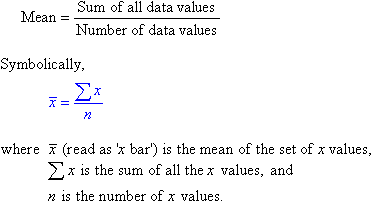### Java Program To Calculate Average Of N Numbers

How to calculate the average of N Numbers?
The calculation of the average of N numbers ins quite simple: Here is the formula for it. Hope you get to understand-The Average has been calculated as the sum of all data values / Number of data values.
class Average
{
public static void main(String arg[])
{
int n=5,result=0;

int a[]=new int;

a=10;

a=20;

a=30;

a=40;

a=50;

for(int i=0;i<n;i++)
result=result+a[i];

System.out.println("average of  ("+a+","+a+","+a+","+a+","+a+")  is ="+result/n);

}
}
output:-
average of(10,20,30,40,50) is=30

2. Taking inputs through scanner class

java.util.Scanner;
class Average
{
public static void main(String arg[])

{

int n;double res=0;

Scanner sc=new Scanner(System.in);

System.out.println("enter how many numbers to cal  avg");

n=sc.nextInt();

int a[]=new int[n];

System.out.println("enter   "+n+"  numbers");

for(int i=0;i<n;i++)
a[i]=sc.nextInt();

for(int i=0;i<n;i++)
res =res+a[i];

System.out.println("average="+res/n);

}

}

Output:-

enter how many numbers to cal  avg
6
enter 6 number
1
2
3
4
5
6
average=3.5